# Using GEBS Reporting Excel Converter

This current use case will show the manner in which the data from data source shall get to the Excel output file. The data source is represented here by a Rational DOORS module.An object in the DOORS module has plenty of properties. Here we’re interested especially about the numerical ones.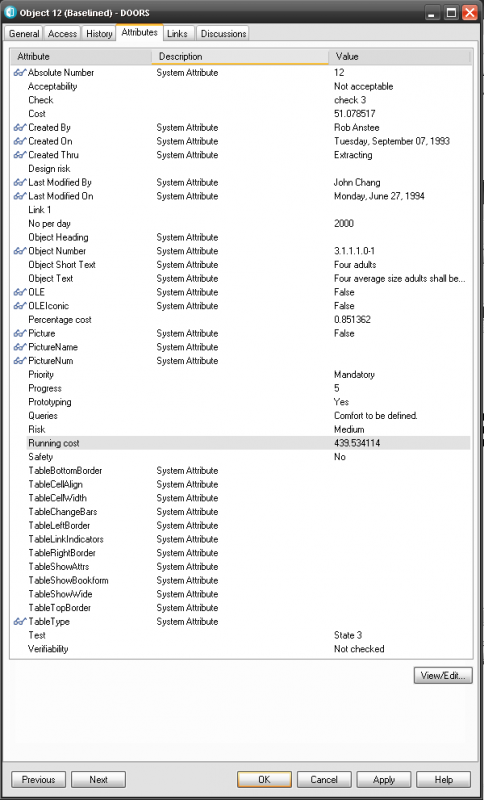By running schema discovery in RPE, we can get the object attributes that we’re interested to have in the final Excel output. These attributes shall be first extracted in the XslFo output with the help of the following RPE template: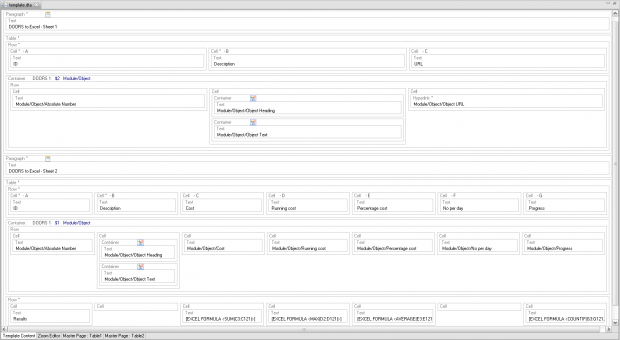The template contains two masterpages. Every masterpage will generate a new sheet in Excel. There are some formulas in the last row of the 2nd table, starting with the 3rd cell :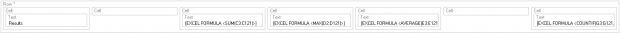[EXCEL FORMULA ] calculates the sum of all “Cost” values within current column :[EXCEL FORMULA ] calculates the maximum value from the “Running cost” column :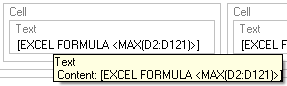[EXCEL FORMULA ] calculates the average for all the values in the “Percentage cost” column :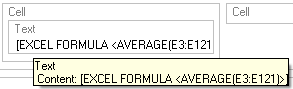[EXCEL FORMULA ] calculates the number of cells that have “0” as value, to check out the status of the “Progress” column :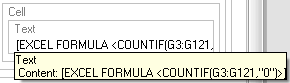After running the template, the XslFo output shall have the structure that Excel Convertor can recognize and handle. This is the XslFo section with the Masterpage named “Table1” :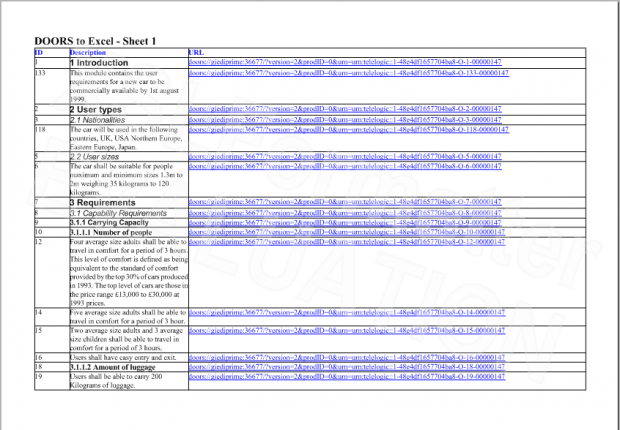This is the XslFo section with the Masterpage named “Table2”: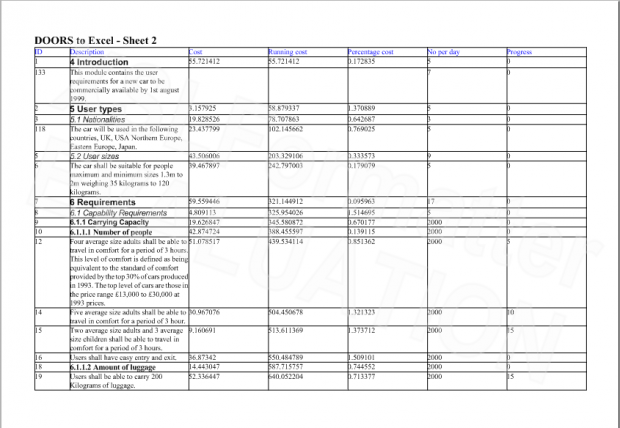..and the last row in 2nd table contains the formulas :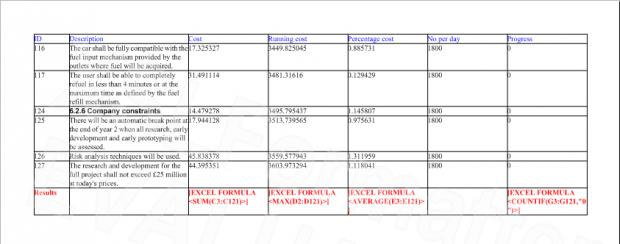The user opens the Excel Convertor tool, selects the XslFo file path and types or selects the Excel output file path. It makes sure that the “Open result” checkbox is selected, and press the “Convert” button.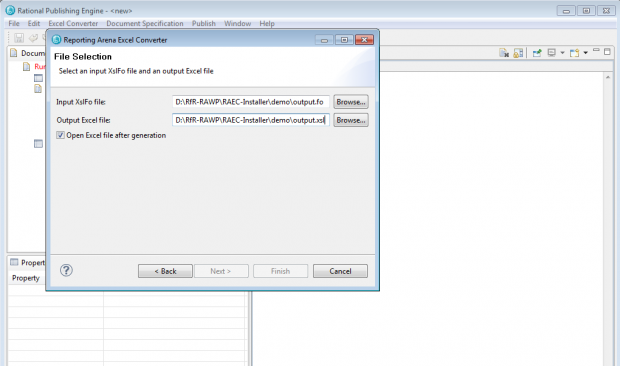The RPE log notifies the user about the status of the converting process. When the process is finished, the excel file opens.
Here is the 1st sheet , called as the masterpage name – “Table1” :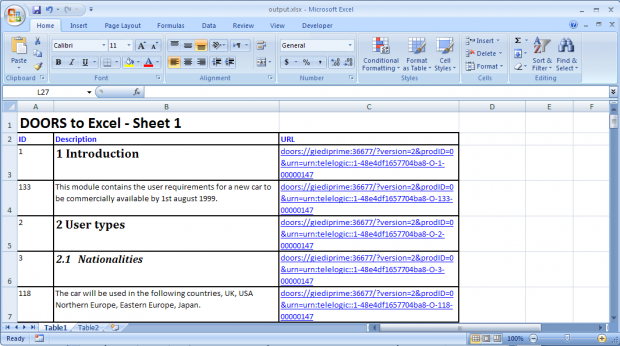Here is the 2nd sheet, named “Table2” :The formulas results will be visible in the last row :